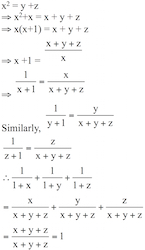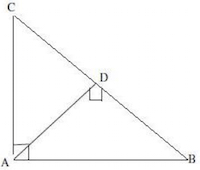# Sample Aptitude Questions of Sail

1. The simple interest on Rs. 4000 in 3 years at the rate of x% per annum equals the simple interest on Rs. 5000 at the rate of 12% per annum in 2 years. The value of x is
1. 8%
2. 9%
3. 10%
4. 6%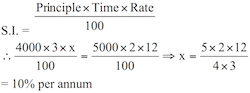2. If x2 – 3x + 1 = 0, then the value of x2 + x + 1/x + 1/x2 is
1. 6
2. 8
3. 10
4. 2

x2 – 3x + 1 = 0
⇒ x2 + 1 = 3x
Dividing both sides by x, => x + 1/x = 33. If (4x-3)/x + (4y-3)/y + (4z-3)/z = 0, then the value of 1/x + 1/y + 1/z is
1. 4
2. 6
3. 9
4. 3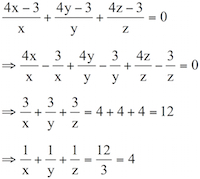4. Find the simplest value of 2√50 + √18 - √72 (given √2 = 1.414)
1. 10.312
2. 8.484
3. 4.242
4. 9.898

Expression5. If a2 + b2 + 4c2 = 2(a + b – 2c) – 3 and a, b, c are real, then the value of (a2 + b2 + c2) is
1. 2
2. 2 1/4
3. 3
4. 3 1/4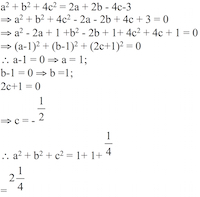6. A number x when divided by 289 leaves 18 as a remainder. Find the remainder when the same number is divided by 17.
1. 3
2. 1
3. 5
4. 2

Here, the first divisor (289) is a multiple of second divisor (17)
∴ Required remainder = Remainder obtained on dividing 18 by 17 = 1. Hence the answer is option 2.
7. An equation of the form ax + by + c = 0 where a ≠ 0, b ≠ 0, c = 0 represents a straight line which passes through
1. (0, 0)
2. (3, 2)
3. (2, 4)
4. None of these

Ax+by+c = 0
When c = 0 ax+by = 0
by = -ax ⇒ y = -a/b x
when x = 0, y = 0 i.e., this line passes through the origin (0,0)
8. The numerator of a fraction is 4 less than its denominator. If the numerator is decreased by 2 and the denominator is increased by 1, then the denominator becomes eight times the numerator. Find the fraction.
1. -4/8
2. 2/7
3. 3/8
4. 3/7

Original fraction = (x-4)/x
In case II,
8(x-4-2) = x+1 ⇒ 8x+48 = x+1
⇒ 7x=49 ⇒ x=7
∴Original fraction
= (7-4)/7 = 3/7
9. If x2 = y + z, y2 = z + x and z2 = x + y then the value of 1/(1+x) + 1/(1+y) + 1/(1+z) is
1. 2
2. 0
3. -1
4. 1